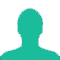# In how many ways can six different rings be worn on four fingers of one hand?

A. 16

B. 24

C. 360

D. 4096

E. 8192

### Solution(By Examveda Team)

Each ring may be worn in any of 4 fingers.
So, each ring may be worn in 4 different ways.
∴ 6 rings may be worm in (4×4×4×4×4×4) = 46 = 4096 ways.

This Question Belongs to Arithmetic Ability >> Permutation And Combination

1.wrong answer.it is solved by permutation not by combination

2.no correct option is there in question.ans is 4^6

3.Wrong hai iska answer 4^6 hoga

Related Questions on Permutation and Combination